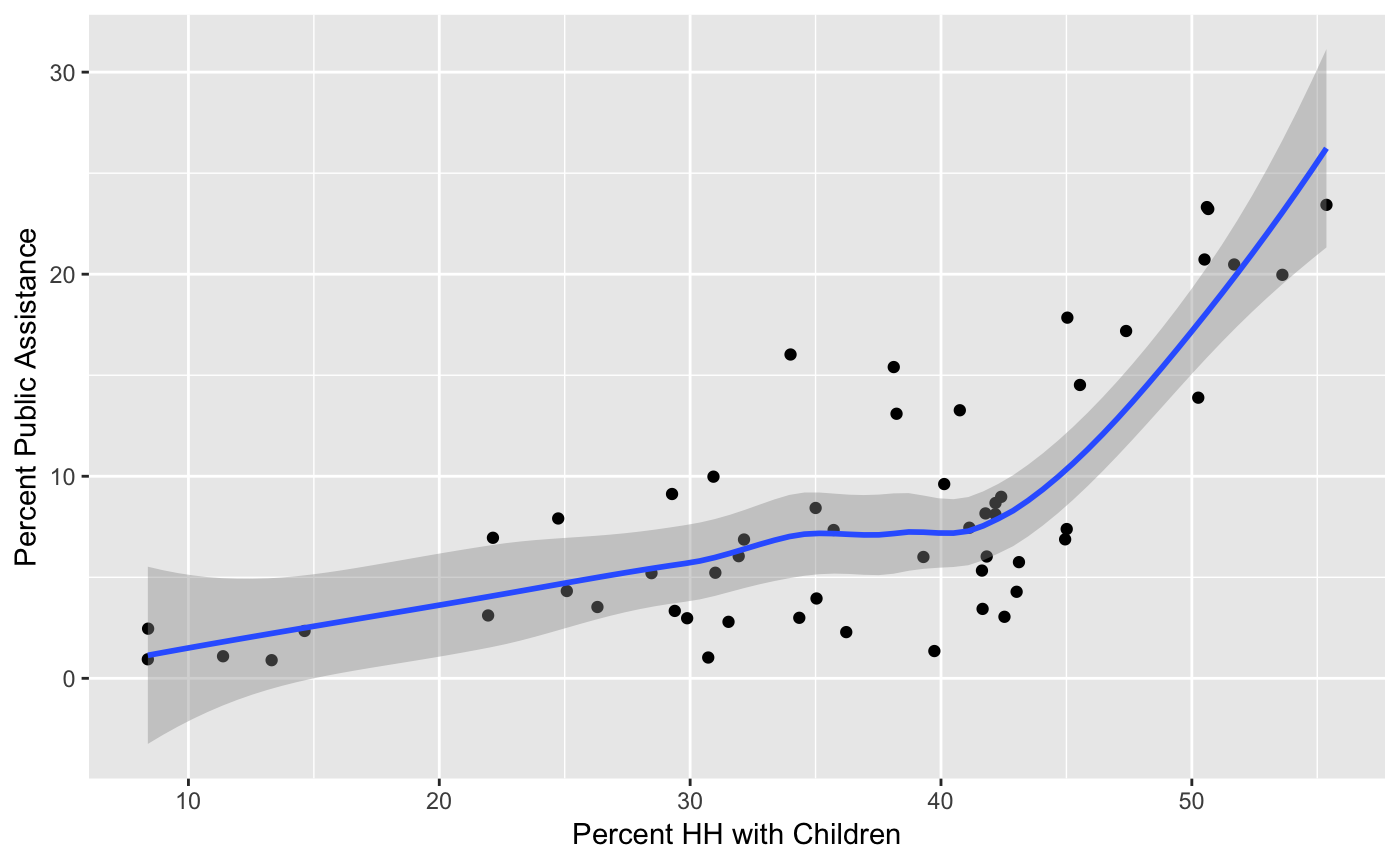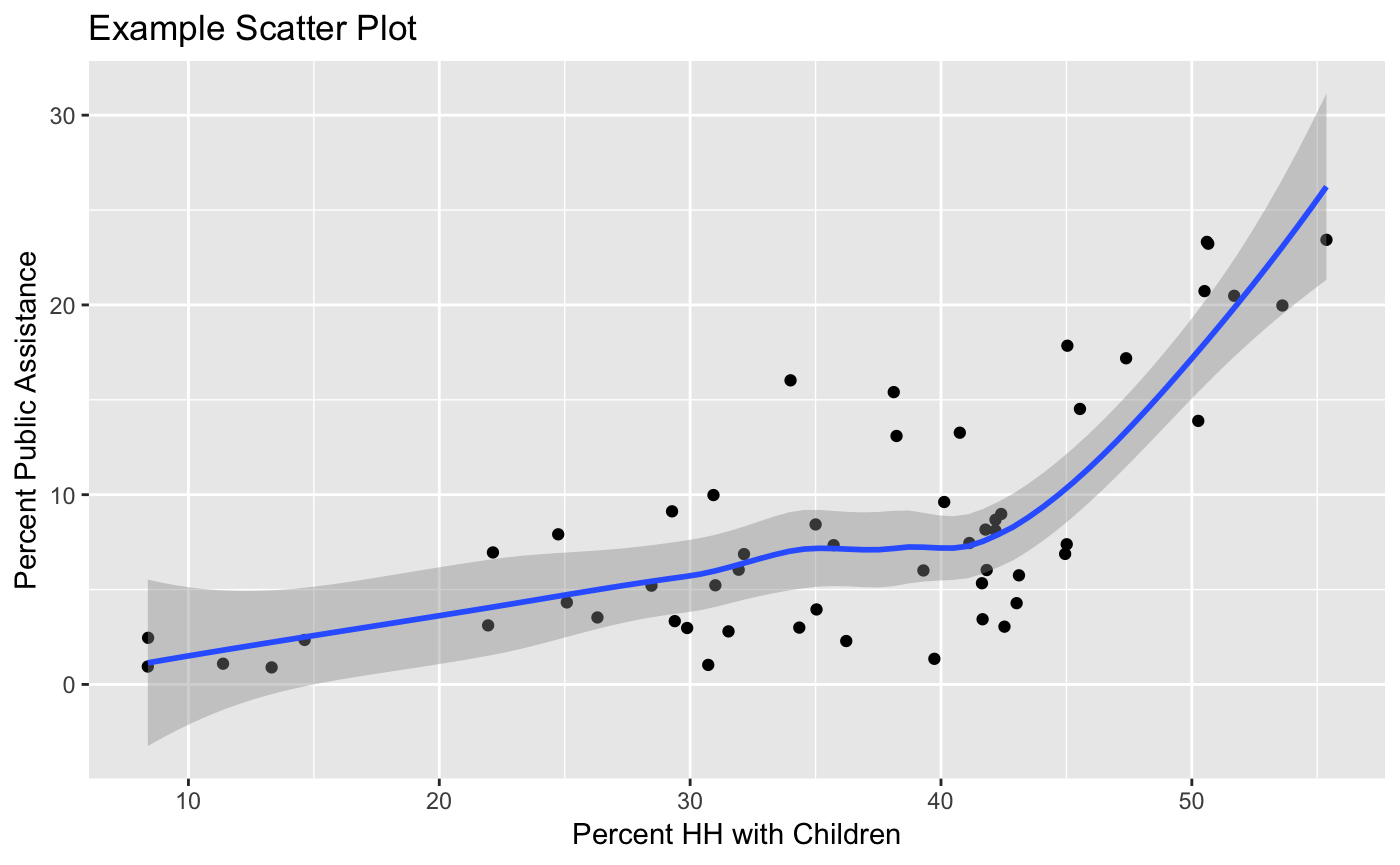## Preliminaries

Make sure to move to the proper working directory and that the file NYC_Sub_borough_Area.dbf is in that directory.

Also, activate the tidyverse and foreign packages using the `library` command (the tidyverse package includes ggplot2):

``````library(tidyverse)
library(foreign)``````

We will continue with the data for the NYC sub-boroughs. If you did not save the data frame from the previous lab, then read it in from NYC_Sub_borough_Area.dbf and add the manbronx variable if you don’t have it. If you saved the data frame using `saveRDS`, then you can load it directly using `readRDS`.

Below follow the commands to start from scratch (check the previous lab for explanations):

``````nyc.data <- read.dbf("NYC_Sub_borough_Area.dbf")
nyc.data <- as_tibble(nyc.data)
nyc.data <- nyc.data %>% mutate(manbronx = if_else((code > 300 & code < 311) | (code > 100 & code < 111),"Select","Rest"))
nyc.data``````

## Spiffing up the Graph

So far, we have used the defaults for the various descriptive aspects of the graph, such as axis labels, title, etc. All these can be specified (in great detail). Only the very basics will be covered here, but the options and combinations are virtually endless.

To illustrate these features, we will continue to use the scatterplot of kids2000 on pubast00, with manbronx as a categorical variable to define subgroups.

### Axis labels

The default setting for the axis labels is to use the variable name. Sometimes, this is not very informative. To set the labels explicitly, we use the `xlab` and `ylab` functions These are added in the same way as actual layers, using the + notation and with the respective options in parentheses (do not use an = sign).

For example, with our default scatter plot, we can set `xlab("Percent HH with Children")` and `ylab("Percent Public Assistance")` (note, the font and font size can be specified as well, but we don’t go that far).

As in the previous lab, we assign the main `ggplot` command to the object g to save us some typing.

``g <- ggplot(data=nyc.data,aes(x=kids2000, y=pubast00))``
``````g + geom_point() +
geom_smooth() +
xlab("Percent HH with Children") +
ylab("Percent Public Assistance")``````### Title

A title is added to the graph by means of the `ggtitle` command. Again, enter the desired title in parentheses and enclosed by quotes. For example, we can add `ggtitle("Example Scatter Plot")` (we also keep the axis labels):

``````g + geom_point() +
geom_smooth() +
xlab("Percent HH with Children") +
ylab("Percent Public Assistance") +
ggtitle("Example Scatter Plot")``````The default is to have the title left-aligned. Often, one may want it centered above the graph. Again, this can be customized. We can override the basic settings in the `theme` command. For example, we adjust the `plot.title` (of course, you need to know what everything is called). Specifically, we set the `element_text` property’s horizontal justification (`hjust`) to 0.5. Specifically, we use `theme(plot.title = element_text(hjust = 0.5))`. This centers the title. The number of other refinements is near infinite and beyond our scope at this point.

``````g + geom_point() +
geom_smooth() +
xlab("Percent HH with Children") +
ylab("Percent Public Assistance") +
ggtitle("Example Scatter Plot") +
theme(plot.title = element_text(hjust = 0.5))``````